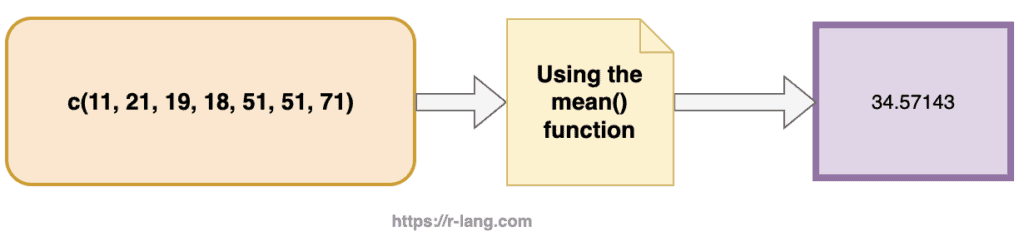# How to Calculate Mean / Average in RTo calculate a mean or average in R, you can “use the mean() function.” For example, mean(c(1, 2, 3, 4)) function returns 2.5.

## Syntax

``mean(x, trim, na.rm)``

## Parameters

1. x: The x is a Numeric Vector.
2. trim: It drops some observations from both ends of the sorted vector.
3. na.rm: It is a boolean value to ignore the NA value.

## Return value

The mean() function returns the arithmetic mean of the input numeric vector.

## Example 1: Calculating the mean of a vector

Using the mean() function to find the mean or average of a vector.

``````rv <- c(11, 21, 19, 18, 51, 51, 71)

# Calculating average using mean()
mean(rv)``````

Output

`` 34.57143``

## Example 2: Calculating the mean of a data frame column

The data frame column is a vector, so you can use the mean() function to calculate the average of the data frame column in R.

``````df <- data.frame(
id = c(11, 22, 33, 44, 55),
price = c(19, 46, 21, 11, 18)
)

# Calculate mean of DataFrame column
mean_of_col <- mean(df\$price)
mean_of_col
``````

Output

`` 23``

## Example 3: Passing the trim option to the mean() function

The mean() function optionally takes the trim parameter. When you pass the trim parameters, the values in the vector get sorted, and then the required observations are dropped from calculating the mean.

If you pass the 0.3, 3 values from each end will be dropped from the calculations to find the mean.

``````# Create a vector.
rv <- c(11, 18, 19, 21, 29, 46)

# Find Mean of rv vector.
meanResult <- mean(rv, trim = 0.3)
print(meanResult)``````

#### Output

`` 21.75``

## Example 4: Ignoring NA values in the mean() function

If there are missing values, then the mean() function returns NA.

``````# Create a vector.
rv <- c(11, 18, 19, NA, 29, 46)

# Find Mean of rv vector.
meanResult <- mean(rv)
print(meanResult)``````

Output

`` NA``

To drop the missing values from the calculation, use na.rm = TRUE. Which means removing the NA values.

``````# Create a vector.
rv <- c(11, 18, 19, NA, 29, 46)

# Find Mean of rv vector.
meanResult <- mean(rv, na.rm = TRUE)
print(meanResult)``````

Output

`` 24.6``

## Example 5: Plotting the mean values using ggplot2

To plot the mean (or average) of a dataset in R, you would typically visualize the data points and then overlay a horizontal line representing the mean value.

``````# Generating 10 random numbers from a standard normal distribution
data <- rnorm(10)

# Calculate the mean
data_mean <- mean(data)

# Plotting the data using base R graphics
plot(1:10, data, pch=19, col="blue", ylim=c(min(data) - 1, max(data) + 1),
xlab="Index", ylab="Value", main="Scatter Plot with Mean Overlay")
abline(h=data_mean, col="red", lwd=2) # Overlaying the mean
grid(col="gray")
legend("topright", legend=paste("Mean =", round(data_mean, 2)), col="red", lwd=2)
``````

Output## Conclusion

Beginner Level: For newcomers, the mean() function is a simple function to calculate averages of numeric value quickly.

Intermediate Level: At this level, understanding how to handle missing values with na.rm or using the trim parameter for a trimmed mean becomes important.

Advanced Level: Advanced users might need to implement weighted means manually or use other specialized packages for robust statistical analysis. They should also be aware of type coercion behaviors when applying mean() to non-numeric vectors.

## Related posts

Standard deviation in R

Variance in R

Standard Error in R